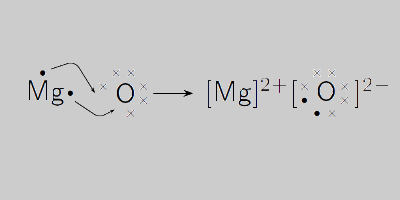# Magnesium Oxide Formula

Magnesium Oxide formula is one of the simple formulas that students can easily memorize and remember during tests or exams. While the formula is given below, let us briefly learn about the compound itself. Magnesium oxide, also known as magnesia is an alkaline earth metal oxide which is usually obtained from the calcination of some naturally occurring minerals. It is a solid that is physically and chemically stable at high temperatures. Thus, it is used mostly in refractory material.

## Magnesium Oxide Formula

Magnesium oxide is made up of Mg2+ and O2- ions and are connected to each other by an ionic bond. However, on looking at the valency we can say that the net charge in the molecules is zero and balanced where we further get one magnesium atom and one oxygen atom. Therefore the formula of Magnesium Oxide is given as MgO.

 Formula MgO Molar Mass 40.3044 g/mol Density 3.58 g/cm³ Melting Point 714 °C Boiling Point 1090°C

### Magnesium Oxide Structural Formula

The magnesium oxide structural formula is represented as;Do stay tuned to BYJU’S to know more about the different formulas of important chemical compounds.